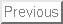Next: Contents

The Pennsylvania State University
College of Engineering

A DISCUSSION OF METHODS OF REAL-TIME AIRPLANE FLIGHT SIMULATION

A paper in
Aerospace Engineering
by
Carl Banks

### Abstract:

This paper discusses the methods of flight simulation; specifically real-time simulation of airplanes. The paper describes methods to form a minimal, but complete, flight simulator. The simulator basically operates by solving the airplane's equations of motion of the airplane in real time. The major part of solving the equations of motion is determining the total force and moment on the airplane. There are many ways to model aerodynamic forces. This paper describes three general models: polynomial modeling, where each resultant force and moment is expressed as a polynomial function; multipoint modeling, where different parts of the aircraft are modeled separately; and tabular modeling, where the forces and moments are looked up in tables. The simulator must also calculate forces and moments produced by the engine and landing gear. The simulator solves the equations of motion in a real-time loop. In each loop, outputs the scenery based on the state, inputs the control positions and settings, calculates the state at the next time step, and finally pauses to synchronize the loop to real time.Next: Contents
Carl Banks
2000-08-11